# Rounding issueHello,

I'm having an issue in which a sum of rounded items is still showing some unexpected decimals.  See screenshot below.  Is there a workaround for this other than using formula as the summary method?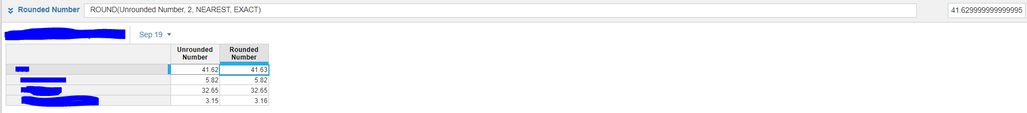Thanks,

Josh

Tagged:

•Hi @jbrass,

This is an interesting issue. Can you describe more of what your goal is here? The way you have it currently is each child member is being rounded, then aggregated up. There appears to be a difference of 0.01.

Few clarifying questions:

1) Can you describe more of what your goal is here?

2) Is this inherent difference problematic? Or is the issue moreso needing further control over the rounding?

3) Are you expecting the unrounded and rounded numbers to be equivilant?

4) Is the issue more specifically focusing on the top right corner which shows the expanded decimal?

-The "formula" summary method would take the unrounded parent and round that number to show in the rounded parent. This would in theory minimize rounding errors as you are rounding only 1 number, instead of rounding 3 numbers then aggregating.

Some ideas come to mind by looking at this at face value (prior to knowing more about your goals):

1. You could have a boolean to drive which numbers get rounded and which numbers don't.

2. You could create a final "number" line item, that would allow you to choose whether you want the rounded number or unrounded number.

3. Maybe a variance measure could help inform which of those numbers you want to take?

Also the number format settings in the blueprint view might help in terms of how many decimal places you want your result to be shown in.

•Also here's some reading material that might spark some thought, I was taking a look at these while formulating my response to you. I know "ROUND" has generally been a FAQ in terms of handling specific scenarios.

https://community.anaplan.com/t5/Anaplan-Platform/Enhancements-for-ROUND/td-p/55053

•The goal is to show a rounded number throughout.  If possible, I am trying to solve this without rounding the aggregated number (using formula as summary method).  That \$.01 (in this example) is problematic, as I'd like for this to be exported to excel and then for a user to be able to sum that data again and get the same end result.   So, to answer #3 below, I do not expect for the rounded and unrounded numbers to be the same (in this case I want the rounded number to be 41.63 while the unrounded number is 41.62).  My goal would be not to build a ton of additional logic to accommodate this.

Given this context, can you provide more insight into your proposed solutions?  For context, let's assume that the row set in question is a million rows (not the 4 row example that I screenshot).

Thanks,

Josh

Thanks,
Josh

•I believe it is because you are looking at the summary member and the number you are rounding is also a formula.  Take a look...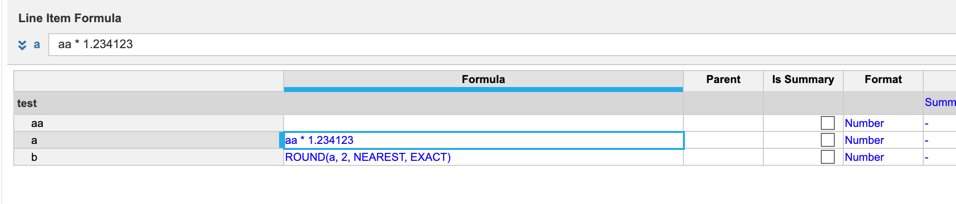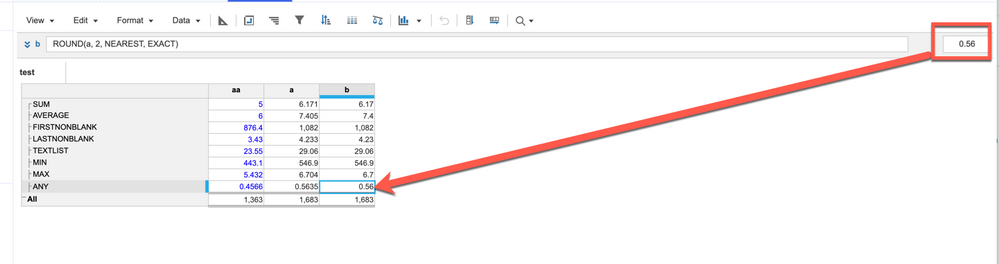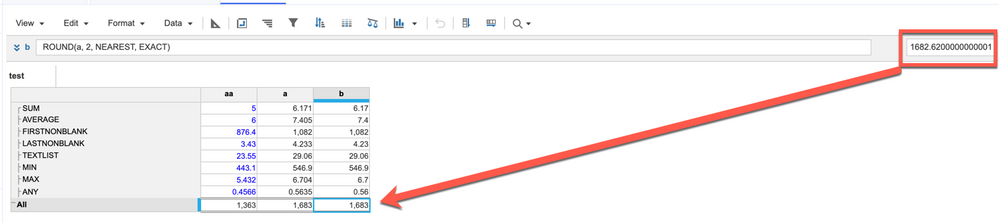But if you change the Summary to Formula, it works: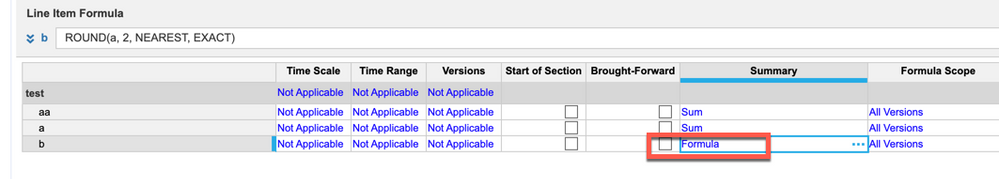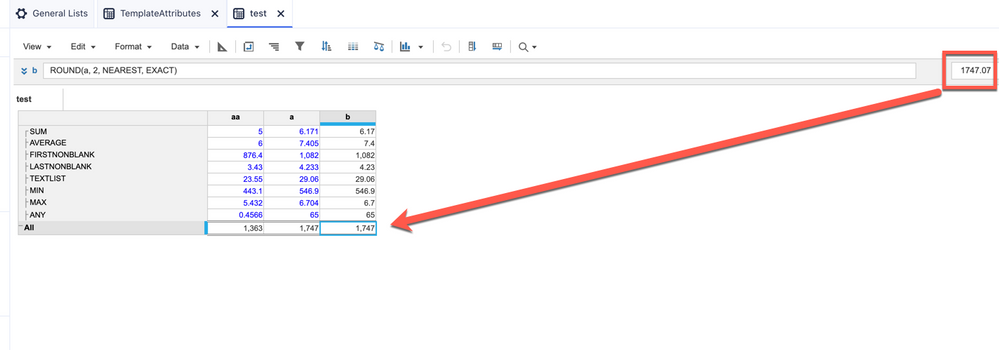Rob

•I just saw the above after my post, you not wanting to use the Summary method of Formula.

•Hi @jbrass ,

I will admit I am still thinking through how to workaround this issue. I did make an attempt to solve this problem, I think I have gotten close. I think I have some groundwork built heading towards a potential solution here, there is perhaps a last piece of logic that is needed here perhaps @rob_marshall you can help finish out the solution.

Preface: I am sort of unsure if we have run up against bug with the Round function itself...or perhaps a limitation. Trying to workaround it may prove to be quite the obstacle....but I figured it is worth a shot to go down the rabbit hole.

So here goes nothing:

1. I created a System Module that breaksdown the Hierarchy levels, and assigns it a number. This is sort of a complex setup, involves changing the applies to column, utilizing ratios, subsidiary views, etc. It is a 1 time setup. Me trying to explain this would do this article an injustice. Highly reccommend checking out how to setup a Hierarchy Level module in that link. I believe this can be useful for our situation here.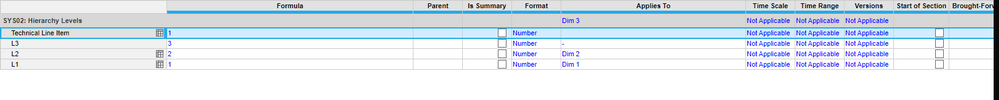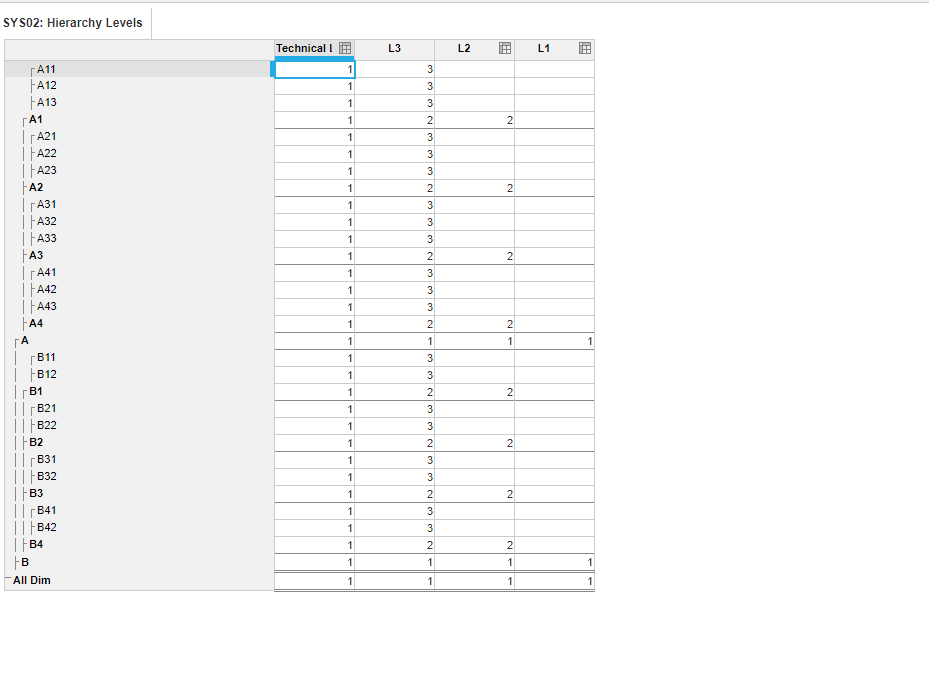2. After I created the Hierarchy Levels Module, I proceeded to then go into the Calculation module where we are trying to do the rounding.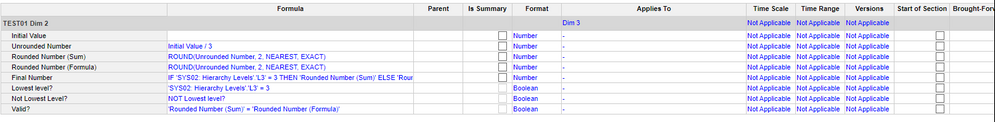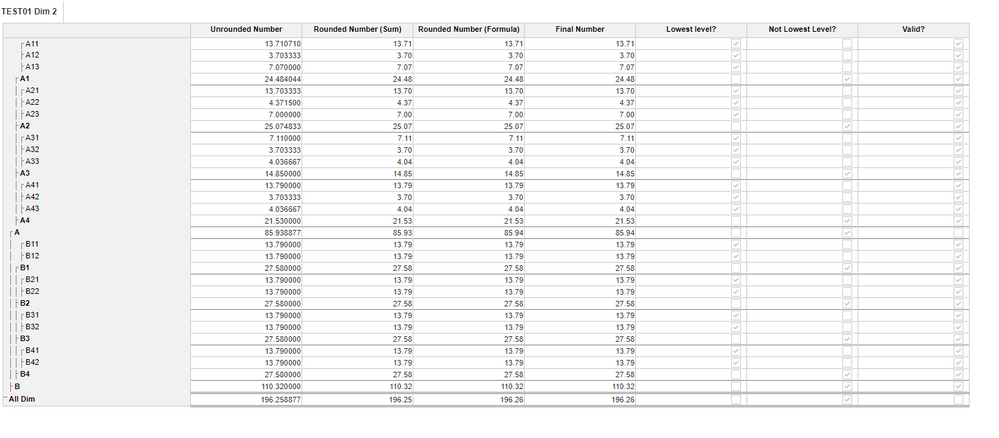3. I went ahead and created 2 identical Rounded Number columns. 1 utilizing summary method of SUM and the other utilizing the summary method of Formula.

4. The "Final Number" column should be of most interest here, as the logic which I have applied states, "if Lowest Level then take the "SUM" version of the rounded number, ELSE take the "formula version" of the number.

Formula: IF 'SYS02: Hierarchy Levels'.'L3' = 3 THEN 'Rounded Number (Sum)' ELSE 'Rounded Number (Formula)'

5. @rob_marshall - this is where I perhaps lean towards you, if you are with me through this point. There is a validation boolean which I have created that does a check to see if Rounded (SUM) = Rounded (Formula). There are some marginal instances where we have invalid scenarios. Do you have any thoughts on how to handle these? Can there be additional logic to handle scenarios where the logic does not hold true?

Maybe add some logic which updates the Final Number formula to this? Final Number = IF NOT VALID THEN 'Rounded Number (Formula)' ELSE IF 'SYS02: Hierarchy Levels'.'L3' = 3 THEN 'Rounded Number (Sum)' ELSE 'Rounded Number (Formula)'

@jbrass - hoping this sparks some thought.

@JaredDolich @Misbah -Going to loop in a few others here, that may be able to carry the ball further down the field. Feel free to chime in here, as this is perhaps the closest approximation to a potential solution I could push towards. Wondering if either of you have encountered a similar situation?

•It may not be fairly that simple as I am showing below but when I have tried to replicate the same original issue, am getting the same values in Input and ROUND calc.

Refer below

Input value as 41.629999999999 in the NUMBER cell with 2 decimal places option (don't miss to refer arrow to see the actual input value)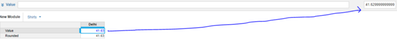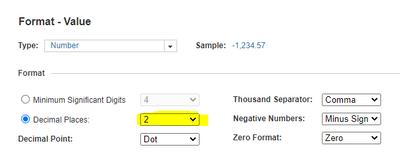(Decimal Places are same in both)

Calc cell with ROUND function: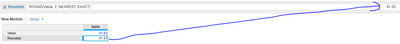Note: Summary method is not changed and kept as SUM (default).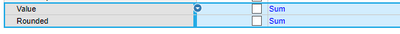Am I missing anything to notice?? since didn't see find issue in input and outcome.

Try out below formula in Calc lineitem once to see if this fixes the issue:

ROUND(Value, 2, TOWARDSZERO)